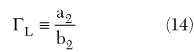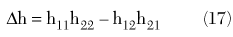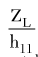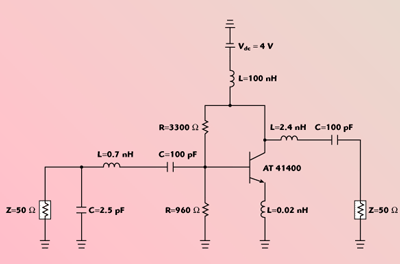There are some fundamental basics on two-port network models. Microwave amplifiers, like the one depicted in the block diagram of Figure 1, have been traditionally designed with scattering parameters with a goal of power gain over some prescribed bandwidth. This model format is justified because, at high frequencies, S-parameters are much more easily and accurately measured than other voltage- or current-based representations. Measuring h-parameters, for example, was a nightmare in the early ’60s because of stability problems using a General Radio 1640 Transfer Function Bridge or a Rohde & Schwarz Z–G Diagraph. When the network analyzer became available for the broadband measurements of S-parameters in around 1965, however, this stability problem was solved, because the broadband 50 ? terminations provided the necessary power dissipations and stability.Fig. 1  Block diagram of a microwave amplifier reperesented as a doubly terminated two-port network whose model is a general k-parameter matrix.

Except for these measurement issues, the various forms of common two-port network parameters are exactly equivalent. Indeed, note that the similarity in format of the definition of several two-port parameters like the impedance, z-; admittance, y-; hybrid, h-; or scattering, S-parameters,andis a natural consequence of the fact that they are all stating the same network characteristic — linearity. Because such a two-input/two-output system is linear, that is, obeys superposition, its outputs — voltages (V1, V2), currents (I1, I2) or reflected waves (b1, b2) — can be given as simple linear combinations of the inputs — currents (I1, I2), voltages (V1, V2) or incident waves (a1, a2) — for z-, y- and S-parameters, respectively. The distinct arrangement of the input and output variables determines that the identification of each of these sets of parameters requires the test of the device under specific boundary conditions: zero currents, or open-circuit terminations, for the z-matrix; zero voltages, or short-circuits, for the y-matrix; a combination of both open-circuits and short-circuits for the h-matrix; and zero incident waves, or perfect matched terminations, for the S-matrix.

The referred equivalence between voltage- and current-based parameters, as the z-, y- and h-matrices, and the incident and reflected waves, as the S- matrix, can even be put in more evidence if some relations are presented to pass from these two distinct modeling domains. Actually, using the basic two-port description, the incident and reflected rms voltage waves can be directly related to the voltages and currents at each port by the following method.1 Starting with the transmission line model, the voltage and current at each port, V1,2 and I1,2, can be expressed as the superposition of the incident, Vinc1,2, and reflected, Vref1,2, voltage waves:Alternatively, adding and subtracting these equations givesTherefore, the incident, a1,2, and reflected, b1,2, power waves, normalized to Z0 — usually assumed real and equal to 50 ? — can be given byIn the same way voltage and current could be related to the normalized incident and reflected power waves, their ratios, impedance or admittance, can also be given in terms of ratios between these normalized incident and reflected power waves, the reflection coefficients. For example, referring again to the basic block diagram,andwhere the input and load reflection coefficients are defined byandThe Role of Voltage and Current Gains in Modern RF and Microwave Amplifier Design

As previously stated, the ultimate goal of a microwave amplifier is to raise the signal power. This is normally achieved in low frequency, multi-stage amplifiers by using large amounts of voltage gain, Av, followed by a current gain, Ai, stage. This leads to the conventional untuned amplifier architecture in which a group of common-source (CS) or common-gate (CG) [FETs] or common-emitter (CE) or common-base (CB) [bipolars] Av blocks is then followed by one or two common-drain (CD) or common-collector (CC) Ai stages. Unfortunately, this strategy fails at high frequencies because the device’s internal parasitic capacitances to ground reduce the input impedance, decreasing, therefore, the voltage gain of the preceding stage, while the series inductors reduce the available current gain. This was circumvented in microwave amplifiers by tuning out these parasitics and providing interstage impedance matching. The result is that all stages now contribute with simultaneous voltage and current gain, providing the desired power gain GP.2,3

Av, Ai, or even other voltage- and current-based gain definitions such as transadmittance, Gm, or transimpedance, Zm, are more readily handled using z-, y-, or h-parameters, while GP is better described via the normalized power wave relations, the S-parameters. It should cause no surprise, then, that the latter have been preferred for the design of RF and microwave amplifiers, while the former were left for low frequency base-band designs. However, the reduction of device parasitics provided by microwave monolithic technologies and the availability of very high gain-bandwidth product devices took the first step in changing this situation. More recently, the need to reduce cost, size and weight in wireless circuits has determined a continued path towards higher levels of integration and lower power consumption. Since it has been recognized that this can only be achieved by eliminating reactive components (it is curious to recognize that, despite the fact that only transistors can contribute to the desired power gain, they occupy incomparably smaller chip area than capacitors or inductors), the tuned amplifier design paradigm has been fast changing towards a more low frequency broadband design orientation, which has created a renewed interest in Av, Ai, Zm and Gm, and thus in the old z-, y- and h-parameters.

In addition, the flexibility provided by modern full-custom RF-CMOS technologies, in which one can select the device geometry (basically the channel width, W, and length, L) quite freely, made these black-box representations questionable in comparison to equivalent circuits where the impact of these changes can be readily accounted for. Under such an equivalent circuit model, devices may be best described by the gain definitions related to the terminal voltage and current ratios. For example, it is well known that BJTs are described by the short-circuit current gain — the hybrid parameter h21 in Equation 3 — defined as ? = IC/IB in the CE configuration, or as a ? IC/IE in the CB configuration. Similarly, the FET is best described by the CS short-circuit transconductance, gm, represented in Equation 2 by the admittance parameter y21.

The first aim of this tutorial article is to review some important relations between these alternative modeling approaches, and thus provide new and useful insights for the various transistor and amplifier gain formats.

Voltage, Current and Power Gain Relations in a Microwave Amplifier

Voltage Gain

Studies of amplifiers usually begin with voltage gain. When the amplifiers are subject to input and output terminations of ZG (?G) and ZL (?L), the voltage gain is easily expressed in terms of h-parameters. Referring to the microwave amplifier prototype, the voltage gain will be defined as the ratio between the actual voltage developed at the load, V2, and the voltage at the amplifier input, V1.

Voltage Gain:From this definition of Av and the constitutive relations of the h-parameters, Equation 3, the voltage gain becomeswhere Dh is the device hybrid-determinant given byNotice that Av is roughly proportional to the short-circuit current gain, h21, scaled by the ratio of load impedance to open-circuit input impedanceBy changing variables from voltage and current to incident and reflected power waves, and thus from impedances to reflection coefficients, Av can also be expressed in terms of S-parameters. Starting by realizing that from Equations 9, 10 and 15, Av can be given byand that,4 from Equation 4from Equation 13and from Equation 14Several books4 have shown that the voltage gain can be finally expressed asThis expression shows that Av is often close to S21, and it is exactly S21 when ?L and S11 are zero.

Current Gain

In this context, the current gain is now defined as the ratio between the output current flowing through the load, I2, and the input current entering the device, I1.

Current Gain:Similarly, the current gain Ai can also be expressed by h-parameters and S-parameters as follows. Starting again by the Ai definition of Equation 20 and the h-parameters formulation of Equation 3, it is straightforward to conclude thatUsing again the S-parameter relations of Equation 4 and the ?in and ?L terminations of Equations 13 and 14which is roughly –S21 when ?L and S11 are both zero.

Operative Power Gain

Operative power gain, or herein simply referred to as power gain, is defined as the ratio between the active power (or real power) delivered by the amplifier to its load and the active power delivered by the source to the amplifiers input. Therefore, since the voltage and current gains have already been obtained, the power gain GP may be calculated aswhich shows that the ratio of ?L = Re{1/ZL} to Gin = Re{1/Zin} and the voltage gain, or the ratio of RL = Re{ZL} to Rin = Re{Zin} and the current gain, determine the power gain GP.

Alternatively, by using Av or Ai expressions of Equations 19 or 22, and the impedance to reflection coefficient relations of Equations 11 and 12, the power gain can be also
expressed in terms of the S-parameters atThe concepts of transadmittance (or transconductance) and transimpedance (or transresistance) gain have application whenever the device’s input and output entities have different natures. For example, because vacuum tubes and solid-state FETs are field effect devices, they are usually described by their transconductance, the ratio between anode or drain current and grid or gate voltage, gm. On the other hand, optoelectronic receivers, for instance, are based on broadband amplifiers whose source is a photo-diode. This is an optoelectronic transducer in which the output current is proportional to the incoming light power. So the referred amplifiers are more conveniently described by the dual of transadmittance, the transimpedance Zm, which is defined as the ratio between the open-circuit output voltage and the available source current.

Thus, two more gains will finally be defined, the transadmittance and the transimpedance, as the following ratios:Transimpedance:Taking the form of Equations 3, 25 and 26, these two gains are easily expressed by y- or z-parameters asStarting with Equations 25 and 26 and using Equations 13 and 14, it is possible to express these transadmittance and transimpedance gains in terms of S-parameters asNote that, when ?L and S11 are both zero, these expressions become simplified to Gm = S21/Z0 and Zm = Z0S21.

Discussions

When amplifiers are designed, the results are usually viewed in terms of the transducer power gain (defined as the ratio between the active power delivered to the load to the available generator power) versus frequency. However, their transistors are usually operated as current gain devices, in case of BJTs, or as transconductance devices, in FETs, at least at low frequencies. In both cases, these intrinsic gains will be converted into voltage gain and current gain through the output termination, and then into power gain.

To illustrate these concepts, and the calculations presented in the preceding section, three illustrating examples of amplifiers will now be described, based on different microwave transistor technologies: a Si BJT-based low noise amplifier (LNA), a medium power amplifier using a GaAs MESFET and finally a GaN HEMT-based power amplifier.

Si BJT-based Low Noise Amplifier

The first example is a small-signal design. It is a typical LNA design, using the AT 41400 BJT at 4 GHz, whose schematic diagram is depicted in Figure 2.6 It was designed to provide a transducer power gain of GT = 11.3 dB with an associated noise figure NF = 4.8 dB.Fig. 2  A typical LNA using an AT 41400 Si BJT at 4 GHz.

Biased for a quiescent point of IC = 9.4 mA and VCE = 4 V, this BJT presents the following set of S-parameters:With these parameters, and a load impedance of ZL = 47.7 + j59.5 ?, the following gains were calculated:This shows the power gain of the amplifier is largely obtained from the voltage gain.

GaAs MESFET-based Medium Power Amplifier

Another example, using the MWT9 GaAs MESFET at 8 GHz in a medium power application, is given in Figure 3. Its transducer power gain is GT = 7.8 dB and it has a one dB compression point of P1dB = 21.8 dBm.Fig. 3  Medium power amplifier using a MWT9 GaAs MESFET at 8GHz.

Biased for a quiescent point of IDS = 59.1 mA and VCE = 6 V, this MESFET can be described by the following set of S-parameters:With these parameters, and a load impedance of ZL = 67.9 + j4.7 ?, the following gains were calculated:GaN HEMT-based Power Amplifier

The third example uses a new GaN HEMT device made by Nitronex and is shown in Figure 4. It is a power amplifier operating at 900 MHz, with GT = 16.9 dB and P1 dB = 33 dBm.7,8Fig. 4  Power amplifier example using a GaN HEMT at 900 MHz.

This power amplifier prototype was implemented in MIC technology, using an RT/Duroid high frequency laminate substrate with an ?r = 10.2. Figure 5 shows a photograph of the implemented amplifier board.Fig. 5  The implemented PA MIC board using a GaN HEMT at 900 MHz.

Biased for a quiescent point of IDS = 29.4 mA and VDS = 20 V, this HEMT presents the following set of S-parameters:With these parameters, and a load impedance of ZL = 22.9 + j3.3 ?, the following gains were calculated:Conclusion

Although amplifiers are built for power gain, modern CMOS RFICs are re-discovering the voltage and current gain approaches followed in low frequency broadband designs. Furthermore, the transistor operation may also be better understood by calculating the voltage gain, the current gain or even the transadmittance or transimpedance gains.
These gain concepts were reviewed in this article, where different expressions were derived in terms of voltage/current parameters (as z-, y-, or h-parameters) and incident and reflected normalized power waves (S-parameters). Three typical microwave amplifier designs were then used as illustration examples, covering various transistor technologies (Si BJT, GaAs MESFET and GaN HEMT) and applications (low noise and power amplifiers).

Acknowledgments

The authors would like to acknowledge Chi-Chung Chien and Francisco Madriz of Santa Clara University for their collaboration.

References

1. G.D. Vendelin, Design of Amplifiers and Oscillators Using the S-Parameter Method, John Wiley & Sons Inc., Somerset, NJ, 1982, pp. 10–11.

2. J. Janssens and M. Steyaert, CMOS Cellular Receiver Front-ends — From Specifications to Realization, Kluwer Academic Publications, Dordrecht, The Netherlands, 2002.

3. N. Izyumov and D. Linde, Fundamentals of Radio, Mir Publications, Moscow, USSR, 1976.

4. G.D. Vendelin, A.M. Pavio and U.L. Rohde, Microwave Circuit Design Using Linear and Nonlinear Techniques, John Wiley & Sons Inc., Somerset, NJ, 1990.

5. G. Gonzales, Microwave Transistor Amplifiers — Analysis and Design, Second Edition, Prentice Hall, Upper Saddle River, NJ, 1997.

6. G.D. Vendelin, A.M. Pavio and U.L. Rohde, Microwave Circuit Design Using Linear and Nonlinear Techniques, Second Edition, John Wiley & Sons Inc., Somerset NJ, 2005.

7. P.M. Cabral, J.C. Pedro and N.B. Carvalho, “New Nonlinear Device Model for Microwave Power GaN HEMTs,” 2004 IEEE International Microwave Symposium Digest, pp. 51–54.

8. P.M. Cabral, J.C. Pedro and N.B. Carvalho, “Nonlinear Device Model of Microwave Power GaN HEMTs for High Power Amplifier Design,” IEEE Transactions on Microwave Theory and Techniques, Vol. 52, No. 11, November 2004, pp. 2585–2592.